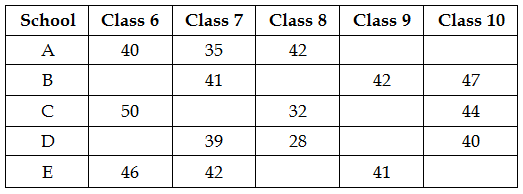## PREPARATORY QUESTIONS

### DATA INTERPRETATION (TABLE)

#### PREPARATORY SET-02 (TABLE)

Direction (Qs.1 to 5): Read the following information carefully and answer the related questions.

Following table represents the data regarding number of students in five schools A, B, C, D and E in different classes.Question No : 1

There are 186 students in class 7 from all schools together and 195 students in class 10 from all schools together. Find the sum of students of class 7 and class 10 from school A and C together if students in class 10 from school A are 24 more than students from school E in same class.

(1) 128

(2) 132

(3) 146

(4) 152

(5) 160

Question No : 2

Students from school A in class 6, 7 and 8 together are approximately what percent of students from school D in same class if sum of students of class 6 and class 8 in school D is 77?

(1) 88%

(2) 92%

(3) 99%

(4) 101%

(5) 107%

Question No : 3

In school C, if there are 40%, 25% and 75% girls in class 6, class 8 and class 10 respectively, find the total number of boys in same class in school C.

(1) 41

(2) 53

(3) 65

(4) 69

(5) 74

Question No : 4

What is the ratio of total students in school D to total students in school E if sum of students of 6th  and 9th class in school D is 101 and sum of students of 8th and 10th class in school E is 9 less than the sum of students of same class in school D?

(1) 11 : 23

(2) 23 : 11

(3) 52 : 47

(4) 47 : 52

(5) 29 : 31

Question No : 5

If number of students in class 7 of school C is the average of total students of class 6th of school A and class 8th of school D Then Find the sum of students in 6th and 7th class from school A and C together?

(1) 135

(2) 142

(3) 159

(4) 164

(5) 167